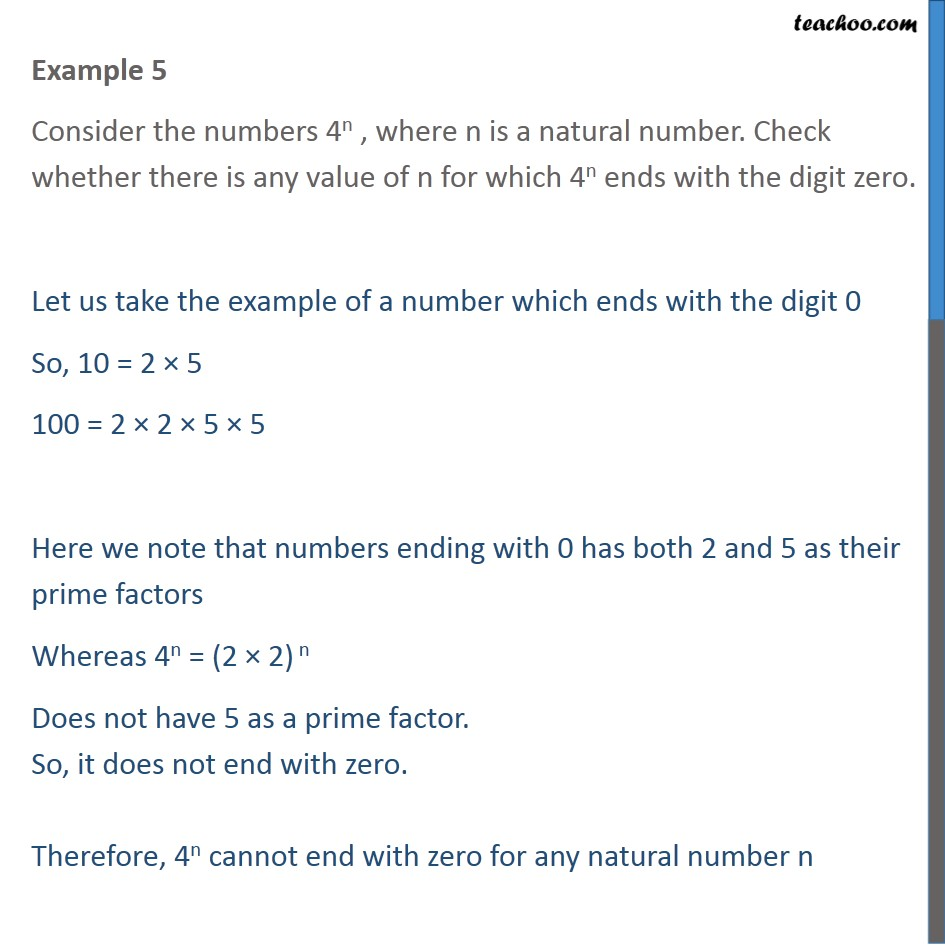Check Important Questions and Chapter Summary -https://you.tube/Real-Numbers-Class-101. Chapter 1 Class 10 Real Numbers
2. Concept wise
3. Prime Factorization

Transcript

Example 5 Consider the numbers 4n , where n is a natural number. Check whether there is any value of n for which 4n ends with the digit zero. Let us take the example of a number which ends with the digit 0 So, 10 = 2 × 5 100 = 2 × 2 × 5 × 5 Here we note that numbers ending with 0 has both 2 and 5 as their prime factors Whereas 4n = (2 × 2) n Does not have 5 as a prime factor. So, it does not end with zero. Therefore, 4n cannot end with zero for any natural number n

Prime Factorization